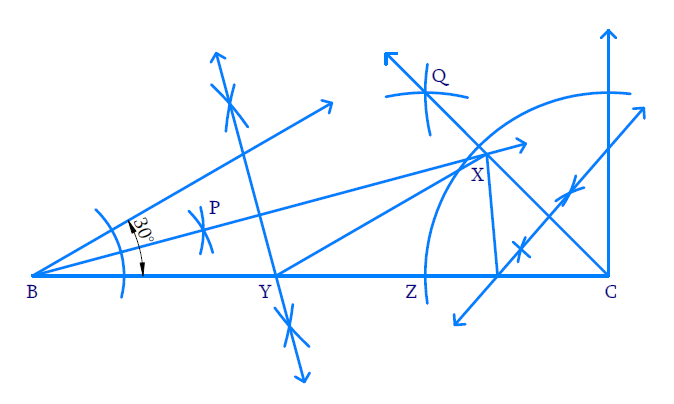In the verge of coronavirus pandemic, we are providing FREE access to our entire Online Curriculum to ensure Learning Doesn't STOP!

# Ex.11.2 Q4 Constructions Solution - NCERT Maths Class 9

Go back to  'Ex.11.2'

## Question

Construct a triangle $$XYZ$$ in which $$\angle Y=30^{\circ}, \angle Z=90^{\circ}$$ and $$XY + YZ + ZX = 11 \;\rm{cm.}$$

Video Solution
Constructions
Ex 11.2 | Question 4

## Text Solution

Steps of Construction:(i) Draw line  $$BC=11\;\rm{cm}$$.

(ii) Make angle of $$30^\circ$$ at $$B$$ and $$90^\circ$$ at $$C$$ using a protractor.

(iii) Bisect angle $$B$$.

With $$B$$ as centre and any radius draw a wide arc to intersect both the arms of angle $$B$$.

(iv) With intersecting points as the centre and same radius draw two arcs to intersect each other at $$P$$ Draw line joining $$B$$ and $$P$$ and extend it beyond $$P$$

(v) Bisect angle $$C$$.

With $$C$$ as the centre and radius draw two arcs to intersect each other at $$Q$$. Join $$Q$$ and $$C$$such that it intersects ray $$BP$$ at $$X$$.

(vi) Perpendicular bisector of $$BX$$.

With $$B$$ and $$X$$ as centres and radius greater than half of $$BX$$ draw arcs on either side of line $$BX$$ to intersect each other. Join the intersecting lines such that the perpendicular bisector intersects $$BC$$ at $$Y$$.

(vii) Perpendicular bisector of $$CX$$

With $$C$$ and $$X$$ as centres and radius greater than half of $$CX$$ draw arcs on Join the intersecting lines such that the perpendicular bisector intersects $$BC$$ at $$Z$$.

(viii) Join $$XY$$ and $$XZ$$. $$XYZ$$ is the required triangle.

Learn from the best math teachers and top your exams

• Live one on one classroom and doubt clearing
• Practice worksheets in and after class for conceptual clarity
• Personalized curriculum to keep up with school